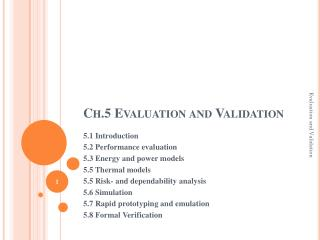DownloadDownload PresentationCh.5 Evaluation and Validation

# Ch.5 Evaluation and Validation

Download Presentation## Ch.5 Evaluation and Validation

- - - - - - - - - - - - - - - - - - - - - - - - - - - E N D - - - - - - - - - - - - - - - - - - - - - - - - - - -
##### Presentation Transcript

1. Ch.5 Evaluation and Validation Evaluation and Validation 5.1 Introduction 5.2 Performance evaluation 5.3 Energy and power models 5.5 Thermal models 5.5 Risk- and dependability analysis 5.6 Simulation 5.7 Rapid prototyping and emulation 5.8 Formal Verification

2. 5.1 Introduction 5.1.1 Scope • Def: Validation is the process of checking whether or not a certain design is appropriate for its purpose, meets all constraints and will perform as expected • Def. Validation with mathematical rigor is called (formal) verification. • Def. Evaluation is the process of computing quantitative information of some key characteristics of a certain design. 5.1.2 Multi-objective optimization • Design evaluation will, in general, lead to a characterization of the design by several criteria, such as average and worst case execution time, energy consumption, code size, dependability and safety. • Finding a set of designs among the designer can then select an appropriate design is the purpose of multi-objective optimization techniques. Evaluation and Validation

3. In order to perform multi-objective optimization, we do consider an m-dimensional space X of possible solution of the optimization problem. • For the space X, we define an n-dimensional function 5.1.3 Relevant objectives • For embedded and cyber-physical systems, multiple objectives need to be considered. • Average performance • Worst case performance/real-time behavior • Energy/power consumption • Temperatures/thermal behavior • Reliability • Numeric precision • Testability • Cost • Weight, robustness, usability, extendibility, security, safety, environmental friendliness Evaluation and Validation

4. 5.2 Performance evaluation 5.2.1 Early phases • Estimated cost and performance values • Accurate cost and performance values 5.2.2 WCET estimation • Scheduling of tasks requires some knowledge about the duration of task executions, especially if meeting time constraints has to be guaranteed, as is in real-time (RT) systems. • The worst case execution time (WCET) is the basis for most scheduling algorithms. Evaluation and Validation Distribution of execution time BCET WCET t BCETEST WCETEST

5. A value analysis computes enclosing intervals for possible values in registers and local variables. The resulting information can be used for control-flow analysis and for data-cache analysis. • The next step is cache and pipeline-analysis. Evaluation and Validation

6. 5.2.3 Real-time calculus • Thicle’s real-time calculus (RTS) is based on the descriptionof the rate of incoming events. This description also includes fluctuations of this rate. • The timing characteristics of a sequence (or stream) of events are represented by a tuple of arrival curves: • Arrival curves periodic stream (left), periodic stream with jitter J (right) Evaluation and Validation 3 2 1 3 2 1 p 2p 3p  p 2p 3p  p-J p+J

7. 5.3 Energy and power models • Energy models and power models are essential for evaluating the corresponding objectives. • The energy E for a certain application is closely related to the power P required per operation, since • Such models are needed for optimization aiming at a reduction of power and energy consumptions. • They are also required for optimizations trying to reduce operating temperatures. • Power estimation is used in power management algorithms. 5.4 Thermal models • The thermal behavior of embedded systems is closely linked to the transformation of electrical energy into heat. Evaluation and Validation

8. 5.5 Risk- and dependability analysis • Definitions: • “A service failure, often abbreviated here to failure, is an event that occurs when the delivered service of a system deviates from the correct service. … A service failure is a transition from correct service to incorrect service.” • An error exists if one of the system’s states is incorrect and may lead to its subsequent service failure. • “The adjudged or hypothesized cause of an error is called a fault. Faults can be internal or external of a system.” • Def.: The Mean Time To Failure (MTTF) is the average time until the next failure, provided that the system was initially working. This average can be computed as the expected value of random variable x: • Def.: The Mean Time To Repair (MTTR) is the average time to repair a system, provided that the system is initially not working. Evaluation and Validation

9. Def.: The Mean Time Between Failure (MTBF) is the average time between two failures. • MTBF is the sum of MTTF and MTTR: MTBF=MTTF+MTTR • Def.: The availability is the probability of a system being in an operational state. 5.8 Formal Verification Evaluation and Validation

10. Evaluation and Validation